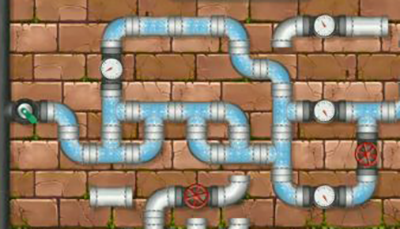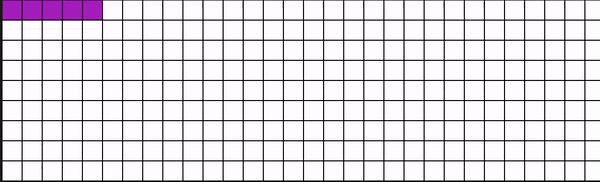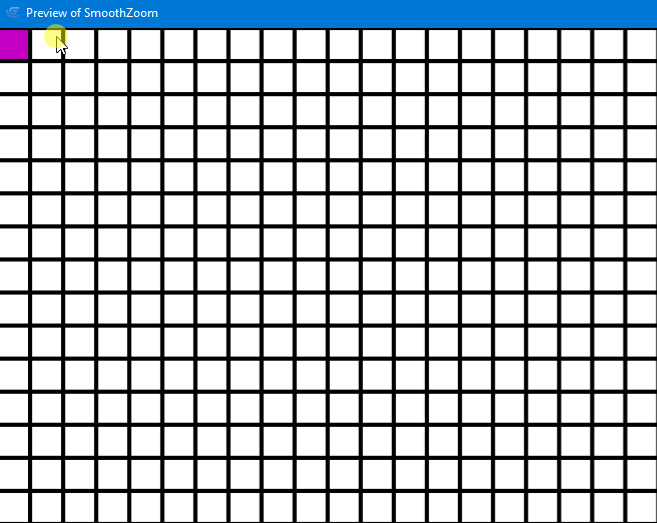# Check if instances are connectedIn my case the rotation of the parts doesn’t matter, but how can I detect for each part if there is a connection with the starting point?
All parts have an id, depending on which position they are in.

Checking next higher and lower id does not work if there is more than one row.

Checking if points from one part are in another part does not work if they are instances of the same object, same with collision.

Many different game mechanics need to check if connections exist. If it’s candy crush or a street in an RTS, but I could never find a solution.

“Checking next higher and lower id does not work if there is more than one row.”

If you add or subtract the number of pieces in a row, you’ll find the higher and lower don’t you?

Then for each different instance shapes, you could have 4 boolean value that indicate if this shape bring to the left, up, to the right and down.

So it could work like a pathfinding, except here you don’t need to calculate the length of the path

Each square of the pathfinding would be either true or false, connected or not with the origin.

Thats just an idea, there might be a better one

Maybe like this.
But e.g. left of id3 is also true if id2 doesn’t exist or is false.
Everything is true as soon as id0 is true.

The main loop would be to constantly look for the square /location / sprite / the variable, whatever we call it, that’s need to be checked. No need to check them all if there’s only 1 variable that is connected to the water.
So first before the loop starts, set the 50 path.n.tocheck to false. Turn the path.startingpoint.tocheck variable to true, where the water comes from.
Also turn path.startingpoint.connected to true
Set n to 1

Then the main loop checking n, adding n at the end of the loop, and if n=51, set n to 1.
Tje condition of the loop is to go on till there is no “to check” variable true anymore. (for that you’ll need a counting variable that count how many times the loop run into a tocheck that is true. When this counter is 0, stop the loop)

Inside the loop:
if path.n.tocheck is true:
higher square:
- if path.(n-50)connected is false
- if direction.up.[path.n.typeofsquare] =true
- and if direction.down.[path.[n-50] .typeofsquare] =true
- - - - - > then set path[n-50]tocheck to true
- - - - - → and set path[n-50]connected to true

left square… And so on

So your main variable would be, for each square n :
Path.n.tocheck
Path.n.connected
Path.n.typeofdquare

And also:
Direction.up.typeofsquare
Direction.left.typefosquare
Direction.right.typefosquare
Direction.down.typefosquare

You have maybe 10 or 11 different types of squares. Each has 4 boolean value allowing or not the 4 directions. This are datas for the full game, since the directions of a type of square doesn’t change.

But on the other hand, according to which square is there in n, path.n.typeofsquare will have to be defined.

I would look at this problem by considering each pipe part as a node in a map. You then use graph theory to determine if two nodes are connected.

@Bluzima @MrMen Many Thanks but that’s way toofor me.

I found a solution using a grid of instances of another object.
(that I already have for other functionalities)
It works when I build a path, but when I leave gaps to fill later, it only works partially and has random bugs, it stop filling connected squares correctly. Why?I’ve also tried it with less than 50 repeats, so there shouldn’t be a timing problem?If you’re using a grid with cells, I’d use object variables and a sprite to check for cell connections, like this :Cell object variables :